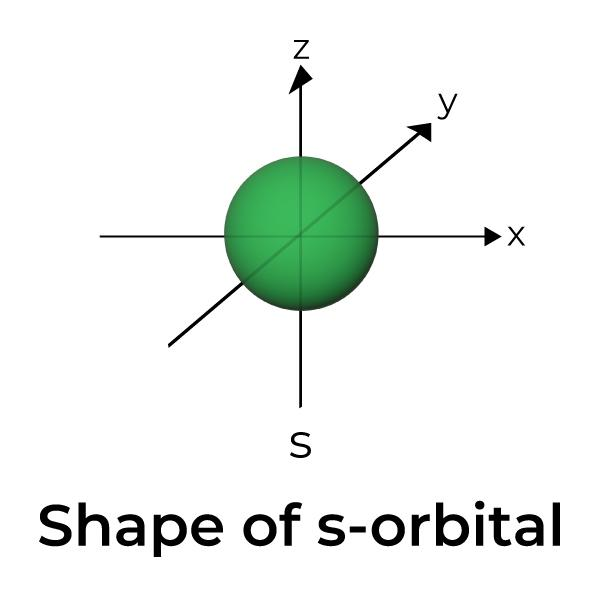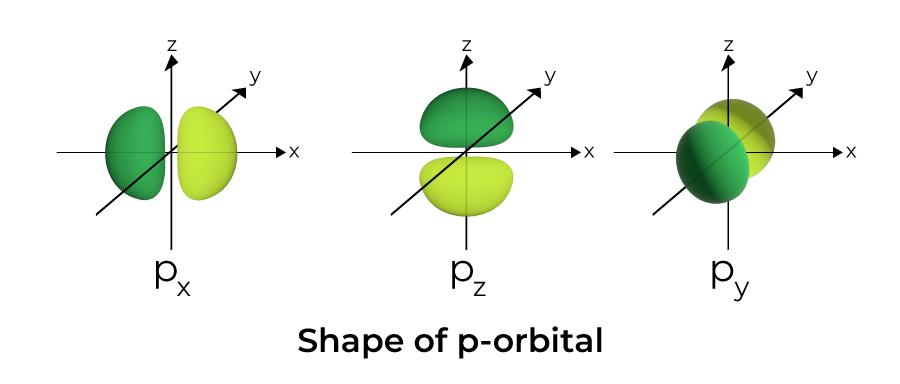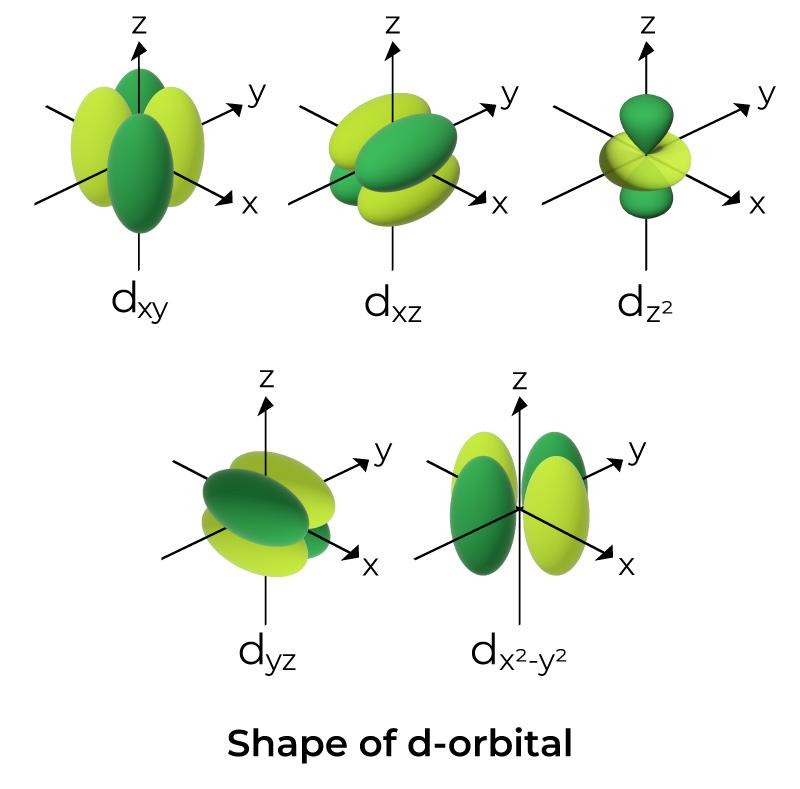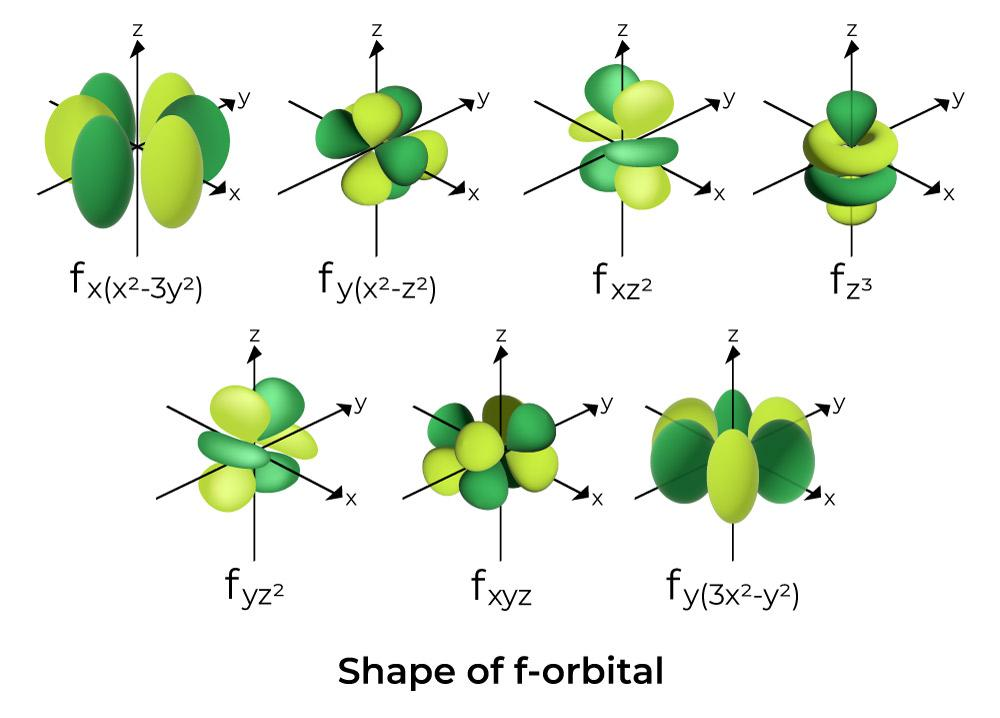GeeksforGeeks App
Open AppBrowser
Continue

## Related Articles

• CBSE Class 11 Chemistry Notes

# Shapes of Atomic Orbitals

Atomic orbitals, which are mathematical functions, describe the wave behavior of electrons (or electron pairs) in an atom. Atomic orbitals have different shapes according to their type. The different shapes of atomic orbitals describe that there is either the highest or zero probability of finding an electron around the nucleus in some directions. There are four types of orbitals, each with a different shape and represented by the letters s, p, d, and f. The s and p orbitals are taken into account because they are the most abundant in chemical and biological chemistry. An s-orbital has a spherical nucleus in the center, a p-orbital is dumbbell-shaped, and four of the five d orbitals are cloverleaf shaped. The fifth d orbital is fashioned like a long dumbbell with a doughnut in the center. The orbitals of an atom are organized into layers or electron shells.

## What are Atomic Orbitals?

Atomic orbitals are the three-dimensional space near the nucleus of an atom where the possibility of discovering an electron is maximum.

An atom, according to the quantum atomic model, can have an infinite number of orbitals. These orbitals can be classified based on their size, shape, or orientation. A narrower orbital means there’s a better probability of catching an electron close to the nucleus. The orbital wave function is often known as a mathematical function that is used to express the coordinates of an electron. The likelihood of locating an electron is represented by the square of the orbital wave function. This wave function also aids in the creation of boundary surface diagrams.

## Orbital Wave Function

The quantum atomic model predicts that an atom can have a huge variety of orbitals. These orbitals can be grouped according to their size, geometry, or inclination. A reduced orbital size increases the probability of obtaining an electron close to the nucleus. The mathematical function used to represent an electron’s coordinates is called the orbital wave function. The probability of discovering an electron is represented by the square of the orbital wave function.

We may also use this wave function to create boundary surface diagrams. We can better comprehend the form of orbitals by using boundary surface diagrams of the constant probability density for various orbitals.

## Shapes of Atomic Orbitals

The atomic orbitals’ shapes vary. That is to say, the probability distributions of the electrons they describe surrounding the nucleus are different. The fact that the electrons in different orbitals are likely to be located in different areas around the parent nucleus and hence experience the latter’s attraction with varied strengths is one of the reasons why orbitals range in energy. It is astonishing that all orbitals in a given shell of the hydrogen atom have the same energy while having varied forms. This is due to the elimination of various energy-related contributions.

But as soon as there is a second electron, the degeneracy disappears.

### Shape of s-orbital

• The s orbital boundary surface diagram resembles a sphere with the nucleus at its center, which can be shown in two dimensions as a circle.
• s-orbitals are spherically symmetric, which means that the probability of finding an electron at a given distance is the same in all directions.
• The size of the s orbital is likewise shown to increase as the value of the primary quantum number (n) increases; hence, 4s > 3s > 2s > 1s.
• The nodal point is a location where there is no chance of locating the electron. Nodes are classified into two types: radial nodes and angular nodes. The distance from the nucleus is calculated by the radial nodes, while the orientation is determined by the angular nodes.### Shape of p-Orbital

• The p orbitals are formed like dumbbells.
• The p orbital node is located at the nucleus’s center.
• Because of the presence of three orbitals, the p orbital can occupy a maximum of six electrons.
• Each p orbital is made up of two parts known as lobes that are located on either side of the plane that runs across the nucleus.
• Each p orbital has parts known as lobes on either side of the plane that runs across the nucleus. At the plane where the two lobes intersect, the likelihood of finding an electron is nil.
• The three orbitals are known as degenerate orbitals because they have the same size, shape, and energy.
• The sole difference between the orbitals is the orientation of the lobes. Because the lobes are orientated along the x, y, or z-axis, they are given the names 2px, 2py, and 2pz. The formula n –2 is used to calculate the number of nodes.
• Similarly to s orbitals, the size and energy of p orbitals rise as the primary quantum number increases (4p > 3p > 2p).### Shape of d-Orbital

• For d orbitals, the magnetic orbital quantum number is given as (-2,-1,0, 1,2). As a result, we can claim there are five d-orbitals.
• These orbitals are denoted by the symbols dxy, dyz, dxz, dx2–y2, and dz2.
• The forms of the first four d orbitals are similar to each other, which differs from the dz2 orbital, but the energy of all five d orbitals is the same.### Shape of f-Orbital

• The form of the f orbital is dispersed. Because the value of l=3 for the f orbital, the minimal value of the primary quantum number n is 4.
• The equivalent ml values for the f orbital are (-3,–2, –1, 0, +1, +2, +3).
• As a result, there are seven f orbitals for l = 3.## Degenerate Orbitals

Degenerate orbitals are those with the same energy. These orbitals are distinct (they may be oriented differently in space around the atomic nucleus), yet they have the same energy. In the presence of an external field, the degeneracy of the p orbital is unaffected; however, the degeneracy of the f and d orbitals can be broken by applying an external field to the system (either electric or magnetic field).

Few orbitals will have higher energy, while others will have lower energy. Degeneracy will no longer exist in the system. For example, d orbitals are made up of five degenerated orbitals that all have the same energy.

## FAQs on Shapes of Atomic Orbitals

Question 1: How do orbitals work?

An atomic orbital is a mathematical expression that expresses the wave-like behaviour of one electron or a pair of electrons in an atom in atomic theory and quantum mechanics. Each orbital will take up a maximum of two electrons, each with its own amount of spin.

Question 2: What is Nodal Plane?

The plane where the probability of finding an electron is negligible or zero is called the Nodal Plane.

Question 3: How many orbitals are there?

Because the s sublevel has just one orbital, only two electrons can be present. Because the p sublevel comprises three orbitals, a maximum of six electrons can be present. Because the d sublevel comprises 5 orbitals, a maximum of 10 electrons can be present. And each of the four sub-levels has seven orbitals that may hold a maximum of 14 electrons.

Question 4: Which orbitals have the highest energy?

The orbital 1s has the most energy. An electron’s energy is the amount of energy required to get it out of the atom’s electrical bubble.

Question 5: What is the difference between a shell and an orbital?